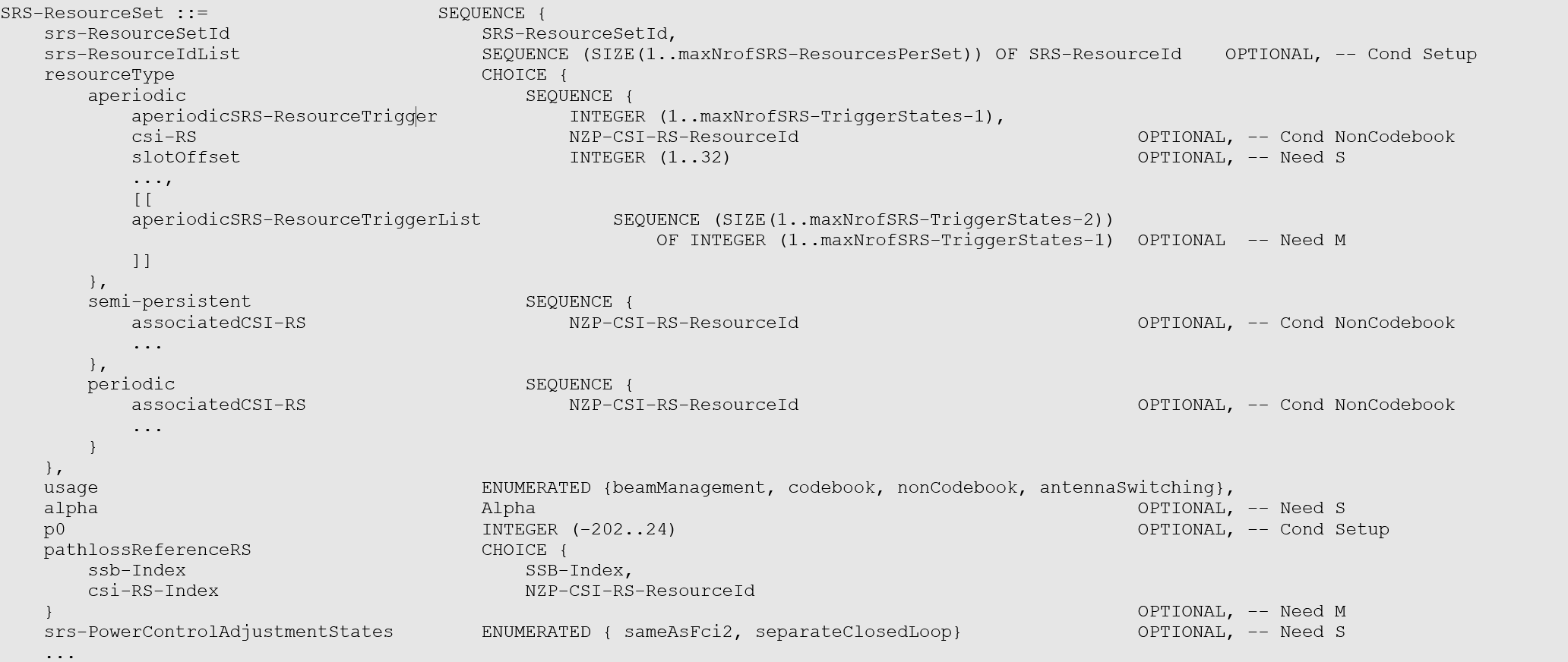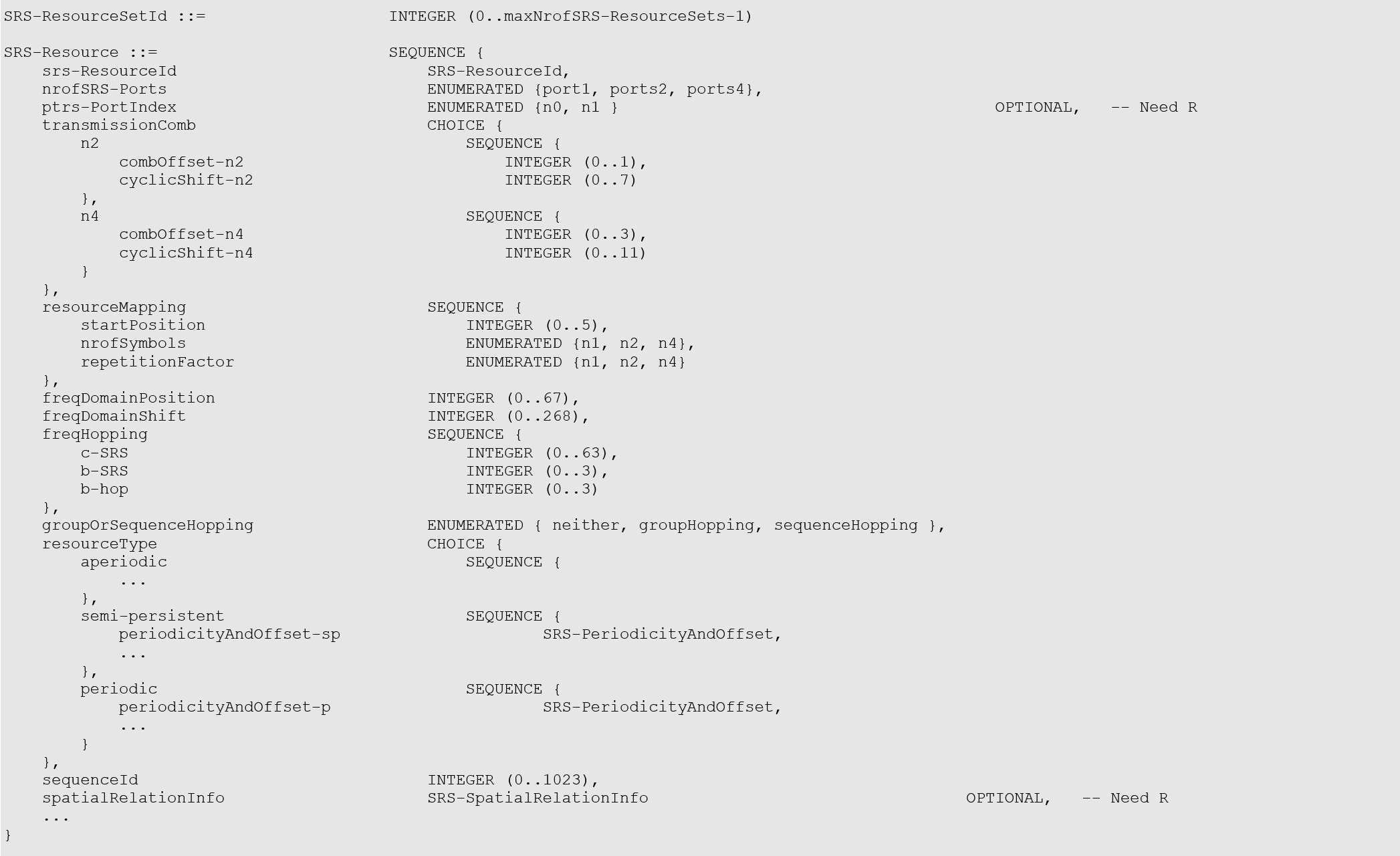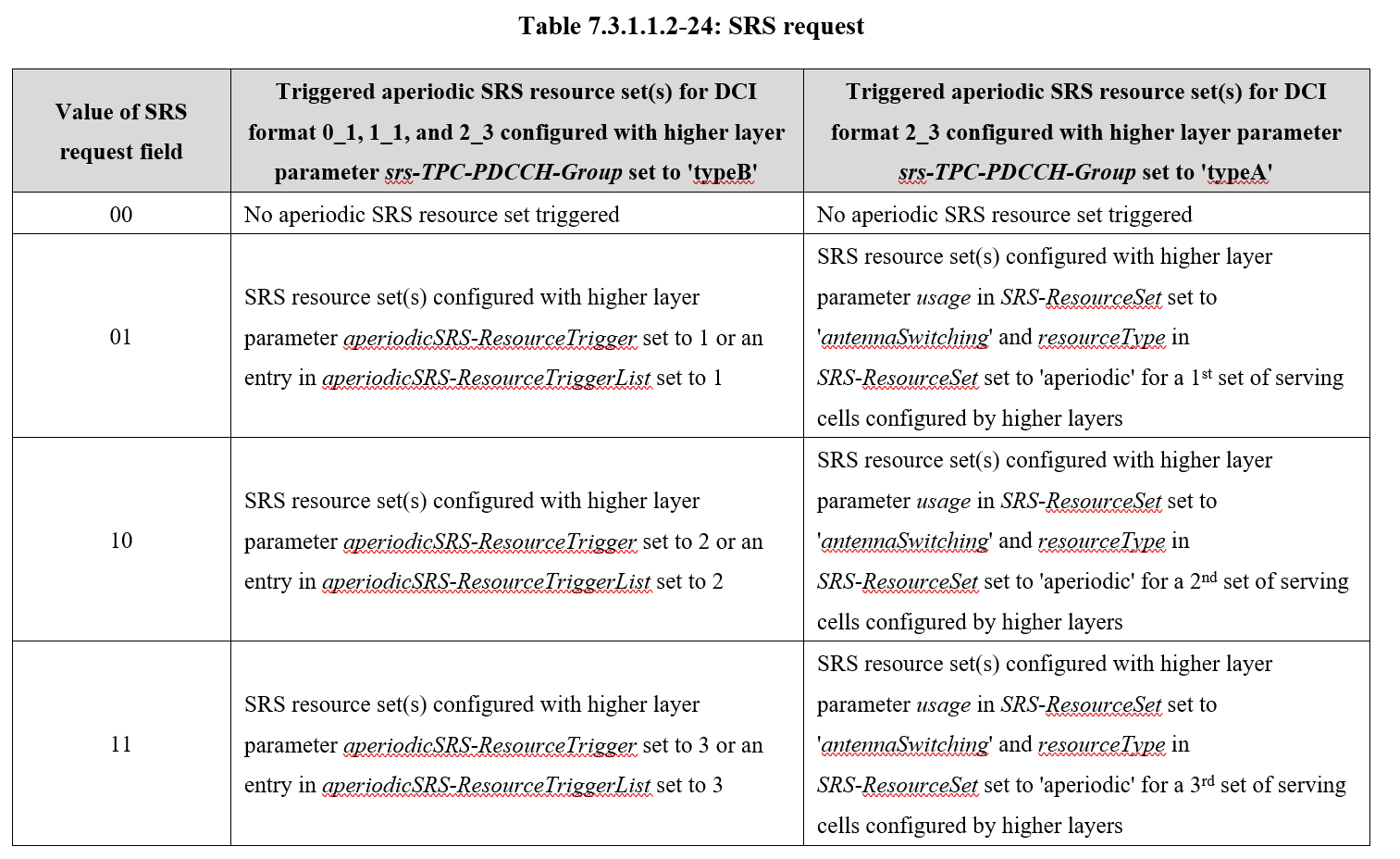0%

Note: 本文基于 3GPP Rel15 (2020-09) 版本规范。

SRS 用于上行信道信息获取、满足信道互易性时的下行信道信息获取以及上行波束管理。

NR 定义了 3 种类型的 SRS 传输：周期性 SRS，半持续性 SRS 和非周期性 SRS，通过为 SRS 资源集和 SRS 资源配置关于时域类型的高层参数 resourceType 来实现。

SRS 资源集配置SRS 资源配置• 周期性 SRS。时域类型被配置为周期 SRS 资源的所有参数由高层信令配置，UE根据所配置的参数进行周期性发送。同一个 SRS 资源集内的所有 SRS 资源具有相同的周期性。考虑到 NR 系统支持各种子载波间隔，不同子载波间隔对应的时隙时长不同，周期 SRS 资源的周期以及周期内的偏移以时隙为单位进行配置。周期 SRS 资源可配置的最小周期为 1 个时隙，最大周期为 2560 个时隙。
• 半持续性 SRS。时域类型被配置为半持续 SRS 资源在激活期间也是周期性发送。它与周期性 SRS 的区别在于 UE 在接收到关于半持续 SRS 资源的高层信令配置后不发送 SRS，只有在接收到 MAC 层发送的关于半持续 SRS 资源的激活信令后才开始周期性地发送半持续 SRS 资源对应的 SRS ，在收到 MAC 层发送的半持续 SRS 资源的去激活命令后停止发送 SRS。因此，相对于周期性 SRS 资源，半持续 SRS 资源的配置以及激活、去激活相比高层信令（RRC信令）更快，更灵活，适用于要求时延较低的业务的快速传输。与周期性 SRS 资源类似，基站通过高层信令为半持续 SRS 资源配置周期和周期内的偏移，同一个 SRS 资源集内的所有SRS资源具有相同的周期性。
• 非周期性 SRS。时域类型被配置为非周期 SRS 资源通过 DCI 信令激活。UE 每接收到一次触发非周期 SRS 资源的 SRS 触发信令，UE 进行一次所触发的 SRS 资源对应的 SRS 发送。DCI 中的 SRS 触发信令包含 2 个比特（如表 Table 7.3.1.1.2-24 所示），2 个比特可表示的 4 个状态。其中中的 1 个状态表示不触发非周期 SRS 发送，其他 3 个状态分别表示触发第一、第二、第三个 SRS 资源组；一个状态可以触发一个或多个 SRS 资源集，一个状态对应的多个 SRS 资源集可以对应多个载波。## PRG

PRG (Precoding Resource Block Group)是预编码资源块组，由一组频域连续的RB组成，这些连续的RB具有相同的预编码。

PRG size的确定涉及到a) UE能力，b) RRC配置，c) UE所调度的连续PRB数目。

### dynamicPRB-BundlingDL

dynamicPRB-BundlingDL 用于指示UE是否支持基于DCI来指示PDSCH接收的PRG size。

dynamicPRB-BundlingDL ENUMERATED {supported} OPTIONAL,

### prb-BundlingType

PDSCH-Config :: prb-BundlingType 用于RRC配置PRG size。

### consecutively scheduled bandwidth in frequency

UE所调度的连续PRB数目将与(BWP size)/2进行比较。

## PRG size

PRG size有{ n2, n4, wideband }三种可能，具体配置策略如下。

1. 如果UE没有dynamicPRB-BundlingDL能力，则gNB只能采用RRC静态配置PRG size。此时prb-BundlingType为staticBundling。
• 如果配置bundleSize = n4，则PRG size = n4
• 如果配置bundleSize = wideband，则PRG size = wideband
1. 如果UE有dynamicPRB-BundlingDL能力，则gNB可采用1）RRC+DCI动态配置PRG size，或2）RRC静态配置PRG size。
• 1）RRC+DCI动态配置，此时prb-BundlingType为dynamicBundling。
• DCI字段PRB bundling size indicator用一个bit来指示bundleSizeSet1或bundleSizeSet2。值为1表示使用bundleSizeSet1，值为0表示使用bundleSizeSet2。
• bundleSizeSet1 = { n4, wideband, n2-wideband, n4-wideband }
• bundleSizeSet2 = { n4, wideband }
• 如果DCI字段PRB bundling size indicator指示bundleSizeSet1
• 如果配置bundleSizeSet1= n4，则PRG size = n4
• 如果配置bundleSizeSet1= wideband ，则PRG size = wideband
• 如果配置bundleSizeSet1= n2-wideband，则
• 如果UE所调度的连续PRB数目 > (BWP size)/2，则PRG size = wideband；否则，PRG size = n2
• 如果配置bundleSizeSet1= n4-wideband，则
• 如果UE所调度的连续PRB数目 > (BWP size)/2，则PRG size = wideband；否则，PRG size = n4
• 如果DCI字段PRB bundling size indicator指示bundleSizeSet2
• 如果配置bundleSizeSet2 = n4，则PRG size = n4
• 如果配置bundleSizeSet2 = wideband，则PRG size = wideband
• 2）RRC静态配置，同a
1. 如有gNB没有配置bundleSize(Set)，则默认PRG size = n2

2. PRG size限制条件

• 如果RBG = 2或vrb-ToPRB-Interleaver = n2，则不能配置PRG size = n4

Note: 当PRG size配置为wideband时，意味着PRG size等于UE所调度的连续PRB数目，分配给UE的资源必须是连续的PRB。在这些连续的PRB上使用相同的预编码。

 3GPP Spec. 38.211  3GPP Spec. 38.212  3GPP Spec. 38.214  3GPP Spec. 38.331  3GPP Spec. 38.306

\begin{align} \mathbf{y=Hx+n} \end{align} (1)

# 预备知识

\begin{align} { {\mathbf{R}}_{x}}=E\left[ \mathbf{x}{ {\mathbf{x}}^{H}} \right] \end{align} (2)

${ {\mathbf{R}}_{n}}=E\left[ \mathbf{n}{ {\mathbf{n}}^{H}} \right] (3)$

${ {\mathbf{R}}_{xn}}=E\left[ \mathbf{x}{ {\mathbf{n}}^{H}} \right]\ ={ {\mathbf{0}}_{ { {N}_{t}}\times { {N}_{r}}}} (4)$

${ {\mathbf{R}}_{nx}}=E\left[ \mathbf{n}{ {\mathbf{x}}^{H}} \right]\ ={ {\mathbf{0}}_{ { {N}_{r}}\times { {N}_{t}}}} (5)$

\begin{align} & { {\mathbf{R}}_{y}}=E\left[ \mathbf{y}{ {\mathbf{y}}^{H}} \right] \\ & \ \ \ \ \ =E\left[ \left( \mathbf{Hx}+\mathbf{n} \right){ {\left( \mathbf{Hx}+\mathbf{n} \right)}^{H}} \right] \\ & \ \ \ \ \ =E\left[ \mathbf{Hx}{ {\mathbf{x}}^{H}}{ {\mathbf{H}}^{H}}+\mathbf{Hx}{ {\mathbf{n}}^{H}}+\mathbf{n}{ {\mathbf{x}}^{H}}{ {\mathbf{H}}^{H}}+\mathbf{n}{ {\mathbf{n}}^{H}} \right] \\ & \ \ \ \ \ =\mathbf{H}E\left[ \mathbf{x}{ {\mathbf{x}}^{H}} \right]{ {\mathbf{H}}^{H}}+\mathbf{H}E\left[ \mathbf{x}{ {\mathbf{n}}^{H}} \right]+E\left[ \mathbf{n}{ {\mathbf{x}}^{H}} \right]{ {\mathbf{H}}^{H}}+E\left[ \mathbf{n}{ {\mathbf{n}}^{H}} \right] \\ & \ \ \ \ \ =\mathbf{H}{ {\mathbf{R}}_{x}}{ {\mathbf{H}}^{H}}+{ {\mathbf{R}}_{n}} \\ \end{align} (6)

# PDCCH

## CCE

PDCCH由一个或多个控制信道单元（control-channel element，CCE）组成，见Table 7.3.2.1-1。

## CORESET

1个CCE由6个资源粒子组（resource-element group，REG）组成，1个REG等于1个OFDM符号上的1个RB。CORESET内的REGs以时域优先的方式按升序编号，从CORESET中第1个OFDM符号且编号最小的RB以0开始编号。

UE可配置多个CORESETs，每个CORESET仅对应一种CCE-to-REG映射。

CORESET中CCE-to-REG映射可以是交织的或非交织的，这由高层参数 CORESET-CCE-REG-mapping-type 来配置，并且由REG捆绑（REG bundle）来描述：

• REG bundle $i$ 定义为一组REGs ${ iL,iL+1,...,iL+L-1 } $$，其中$$ L $$是REG bundle大小，$$ i=0,1,...,{N_{}^{}}/{L};-1 $$，且$$ N_{}{}=N_{}{}N_{}^{}$是CORESET中REG的数目；
• CCE $j$由一组REG bundles ${ f({6j}/{L};),f({6j}/{L};+1),...,f({6j}/{L};+{6}/{L};-1) } $$组成，其中$$ f()$是交织器

\begin{align} \nonumber & f(j)=\left( rC+c+{ {n}_{\text{shift}}} \right)\bmod \left( {N_{\text{REG}}^{\text{CORESET}}}/{L}\; \right) \\ \nonumber & j=cR+r \\ \nonumber & r=0,1,...,R-1 \\ \nonumber & c=0,1,...,C-1 \\ \nonumber & C=\left\lceil {N_{\text{REG}}^{\text{CORESET}}}/{\left( LR \right)}\; \right\rceil \end{align}

• ${ {n}{}}$是物理层小区ID $N{}^{}$ 的函数
• ${ {n}_{}}{ 0,1,...,274 }$是高层参数 CORESET-shift-index 的函数

UE可假定（频域预编码颗粒度）

• 如果高层参数 CORESET-precoder-granularity 等于CORESET-REG-bundle-size，则在REG bundle中，在频域上使用相同的预编码；
• 如果高层参数 CORESET-precoder-granularity 等于CORESET频域大小，则在CORESET中的连续RBs内的所有REGs中，在频域上使用相同的预编码

# PRACH

## 序列生成

\begin{align} \nonumber & { {x}_{u,v}}(n)={ {x}_{u}}((n+{ {C}_{v}})\bmod { {L}_{\text{RA}}}) \\ \nonumber & { {x}_{u}}(i)={ {e}^{-j\frac{\pi ui(i+1)}{ { {L}_{\text{RA}}}}}},i=0,1,...,{ {L}_{\text{RA}}}-1 \end{align}

${ {y}_{u,v}}(n)=\sum\limits_{m=0}^{ { {L}_{\text{RA}}}-1}{ { {x}_{u,v}}(m)\cdot { {e}^{-j\frac{2\pi mn}{ { {L}_{\text{RA}}}}}}}$

# 同步信号（SS）

## 物理层小区ID

1008个唯一的物理层小区ID根据如下公式确定

$N_{\text{ID}}^{\text{cell}}=3N_{\text{ID}}^{\text{(1)}}+N_{\text{ID}}^{\text{(2)}}$